## Excel STANDARDIZE Function

The STANDARDIZE function returns a normalized value (z-score) from a distribution based on the mean and standard deviation of a data set.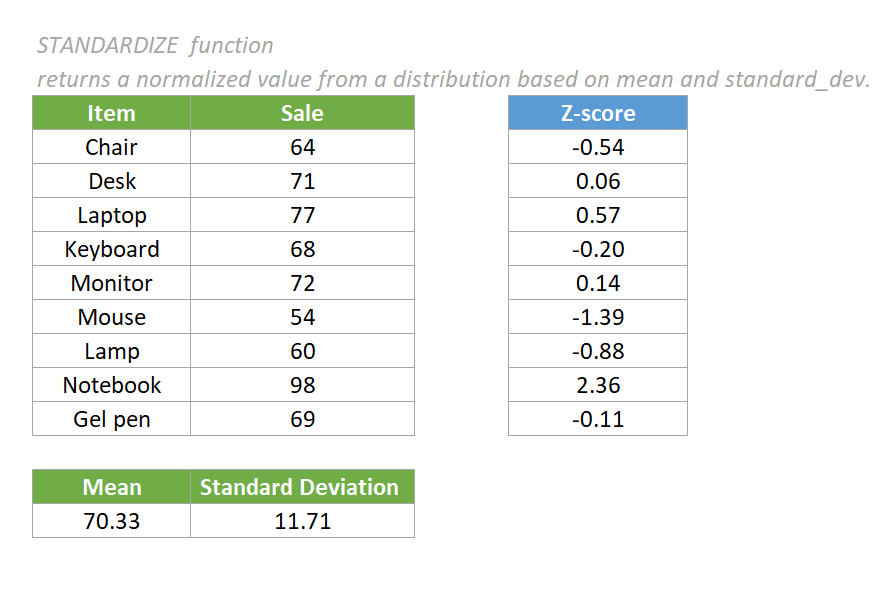#### Syntax

=STANDARDIZE (x, mean, standard_dev)

#### Arguments

• X (required): The value to normalize.
• Mean (required): The arithmetic mean (average) of the distribution.
• Standard_dev (required): The standard deviation of the distribution.

#### Return value

The STANDARDIZE function returns a numeric value.

#### Function notes

1. The equation of the STANDARDIZE function is: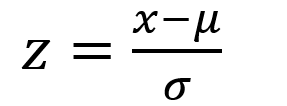2. The #NUM! error value occurs if the supplied standard_dev argument ≤ 0.
3. The #VALUE! error value occurs if any of the supplied arguments are non-numeric.
4. To use the STANDARDIZE function, we need to use the AVERAGE function to calculate the mean and the STDEV.P function to calculate the standard deviation first.

#### Examples

To calculate the normalized value (z-score) from a distribution based on the mean and standard deviation of the values provided in the below table, please do as follows.

1. Use the AVERAGE function to calculate the mean.

Copy the formula below into cell B16, then press the Enter key to get the mean.

=AVERAGE (C5:C13)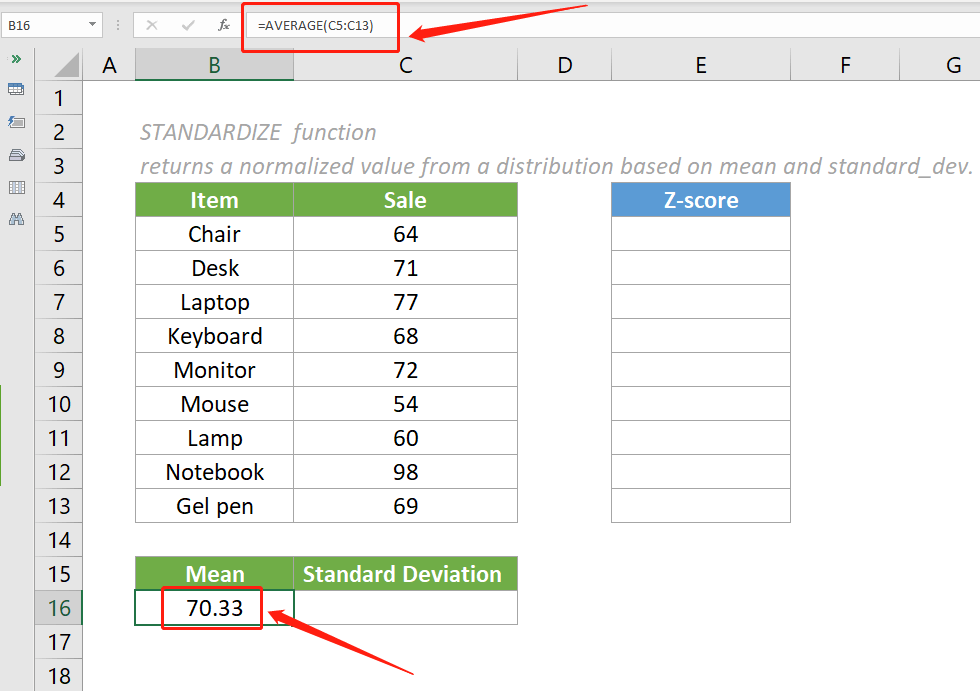2. Use STDEV.P function to calculate the standard deviation.

Copy the formula below into cell B17, then press the Enter key to get the standard deviation.

=STDEV.P (C5:C13)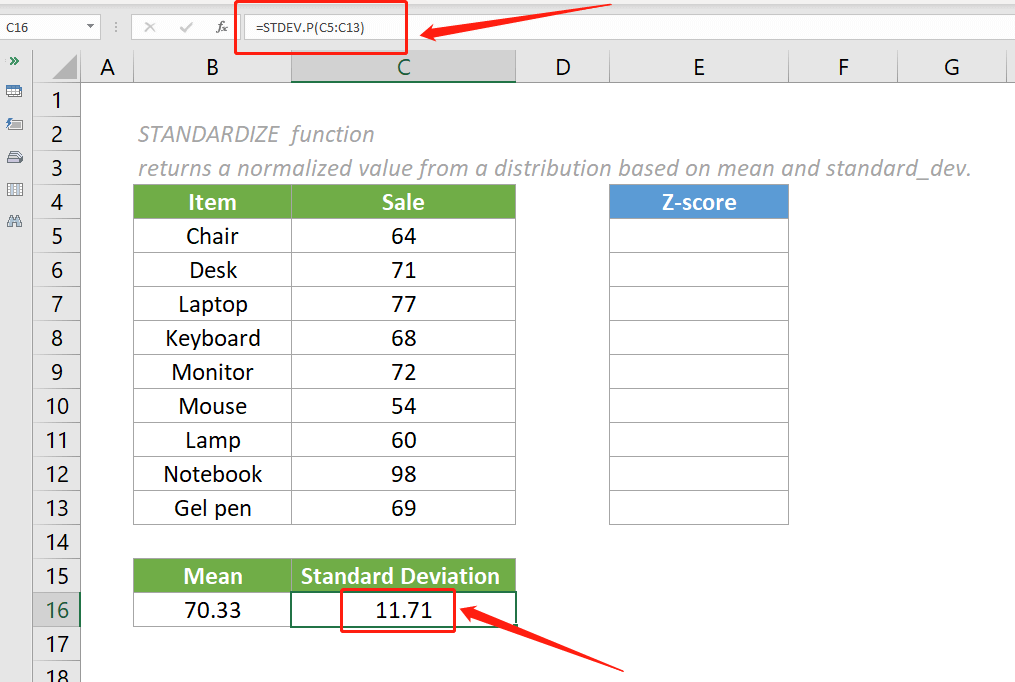3. Calculate the normalized values (z-score) based on the mean and standard deviation in cell B16 and cell C16, using the STANDARDIZE function.

1). Copy the formula below into cell E5, then press the Enter key to get the result.

=STANDARDIZE (C5, B16, C16)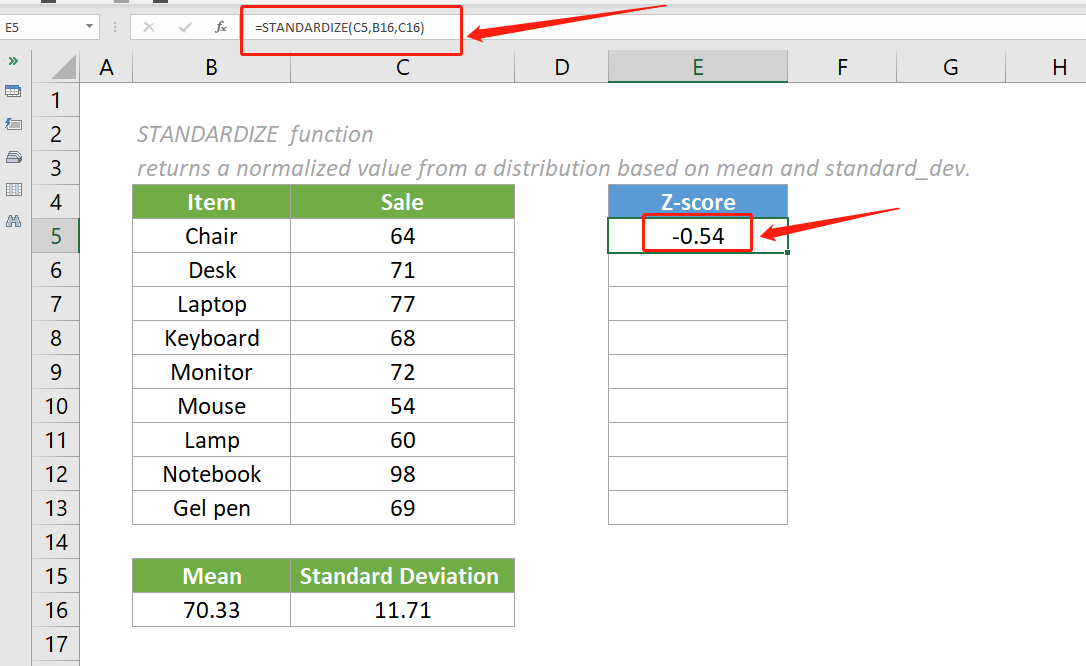2). To get the rest of the results, we need to convert the mean and standard_dev arguments to absolute references in the formula.

In the above formula, select the cell reference B16 and press the F4 key to convert it to an absolute reference. Do the same operation to convert the cell reference C16 to an absolute reference. Then you can see the formula displayed as follows:

=STANDARDIZE (C5, \$B16\$, \$C16\$)

3). Select this result cell and drag its autofill handle down to get the rest of the results.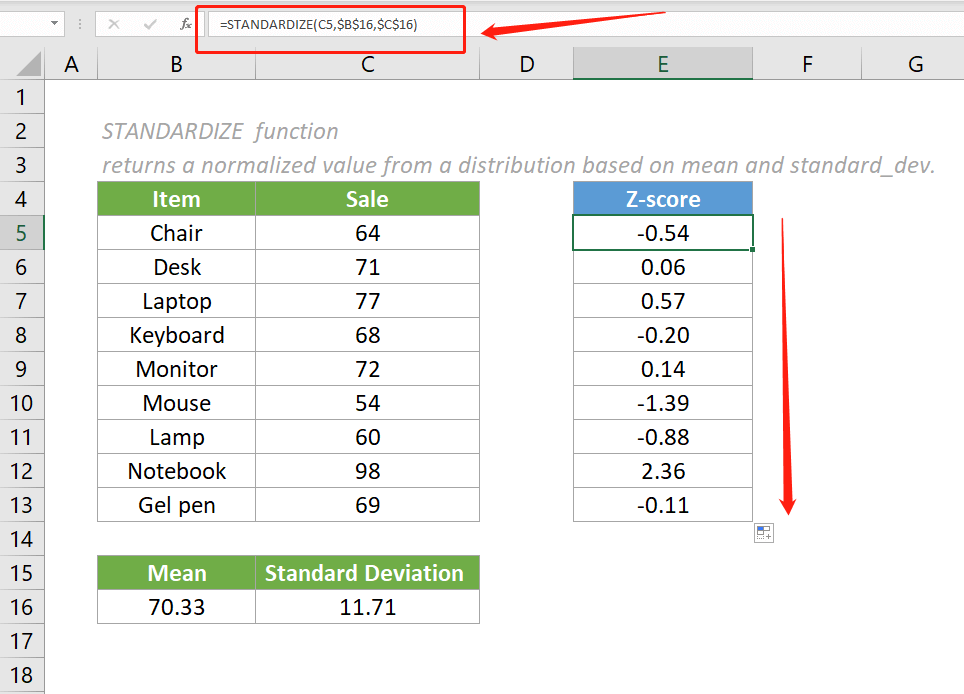Notes:

1. The arguments in the above STANDARDIZE formula are supplied as cell references that contain numeric values.
2. We can also input values directly in the STANDARDIZE formula. For example, the formula in E5 can be changed to:

=STANDARDIZE (64, 70.33, 11.71)

#### Relative Functions:

• Excel EVEN Function
The EVEN function rounds numbers away from zero to the nearest even integer.

• Excel EXP Function
The EXP function returns the result of the constant e raised to the nth power.

### The Best Office Productivity Tools

#### Kutools for Excel - Helps You To Stand Out From Crowd

 Popular Features: Find/Highlight/Identify Duplicates   |  Delete Blank Rows   |  Combine Columns or Cells without Losing Data   |   Round without Formula ... Super Lookup: Multiple Criteria VLookup | Multiple Value VLookup |  VLookup Across Multi-Sheets |  Fuzzy Lookup... Advanced Drop-down List: Easy Drop Down List   |  Dependent Drop Down List   |  Multi-select Drop Down List .... Column Manager: Add a Specific Number of Columns   |   Move Columns   |   Unhide Columns   |   Compare Columns to Select Same & Different Cells ... Featured Features: Grid Focus   |  Design View   |   Big Formula Bar   |  Workbook & Sheet Manager   |  Resource Library (Auto Text)   |  Date Picker   |  Combine Worksheets   |  Encrypt/Decrypt Cells   |  Send Emails by List   |  Super Filter   |   Special Filter (filter bold/italic/strikethrough...) ... Top 15 Toolset:  12 Text Tools (Add Text, Remove Characters ...)   |   50+ Chart Types (Gantt Chart ...)   |   40+ Practical Formulas (Calculate age based on birthday ...)   |   19 Insertion Tools (Insert QR Code, Insert Picture from Path ...)   |   12 Conversion Tools (Numbers to Words, Currency Conversion ...)   |   7 Merge & Split Tools (Advanced Combine Rows, Split Cells ...)   |   Many More...

Kutools for Excel boasts over 300 features, ensuring that what you need is just a click away...

Supports Office/Excel 2007-2021 & newer, including 365   |   Available in 44 languages   |    Full-featured 30-day free trial.#### Office Tab - Enable Tabbed Reading and Editing in Microsoft Office (include Excel)

• One second to switch between dozens of open documents!
• Reduce hundreds of mouse clicks for you every day, say goodbye to mouse hand.
• Increases your productivity by 50% when viewing and editing multiple documents.
• Brings Efficient Tabs to Office (include Excel), Just Like Chrome, Firefox, And New Internet Explorer.